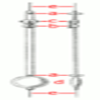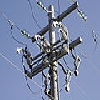# Resources tagged with: 1st and 2nd order linear differential equations with constant coefficients

There are 5 NRICH Mathematical resources connected to 1st and 2nd order linear differential equations with constant coefficients, you may find related items under Calculus.

Broad Topics > Calculus > 1st and 2nd order linear differential equations with constant coefficients### The Not-so-simple Pendulum 2

##### Age 16 to 18Challenge Level

Things are roughened up and friction is now added to the approximate simple pendulum### Differential Equation Matcher

##### Age 16 to 18Challenge Level

Match the descriptions of physical processes to these differential equations.### Phase Space

##### Age 16 to 18Challenge Level

Explore the issues surrounding the concept of Phase Space.### Differential Electricity

##### Age 16 to 18Challenge Level

As a capacitor discharges, its charge changes continuously. Find the differential equation governing this variation.### The Real Hydrogen Atom

##### Age 16 to 18Challenge Level

Dip your toe into the world of quantum mechanics by looking at the Schrodinger equation for hydrogen atoms